# Hands-on ActivityOhm's Law I

### Quick Look

Time Required: 3 hours

Expendable Cost/Group: US \$3.00

\$3 is for light bulbs; the rest of the materials are available in most school classrooms

Group Size: 3

Activity Dependency: None

Subject Areas: Physics, Science and Technology

### Summary

Students experiment to increase the intensity of a light bulb by testing batteries in series and parallel circuits. They learn about Ohm's law, power, parallel and series circuits, and ways to measure voltage and current.

### Engineering Connection

Ohm's law is the basis of all electrical systems. Electrical engineers use this equation to guide the design of electrical systems. Students need a strong foundation in Ohm's law while designing circuits on their own.

### Learning Objectives

Application of the following:

• Ohm's law
• series/parallel circuits (ways to connect them and have an effect on V and I)
• circuit components
• power
• devices that can be used to measure voltage and current

### Educational Standards Each TeachEngineering lesson or activity is correlated to one or more K-12 science, technology, engineering or math (STEM) educational standards. All 100,000+ K-12 STEM standards covered in TeachEngineering are collected, maintained and packaged by the Achievement Standards Network (ASN), a project of D2L (www.achievementstandards.org). In the ASN, standards are hierarchically structured: first by source; e.g., by state; within source by type; e.g., science or mathematics; within type by subtype, then by grade, etc.

###### International Technology and Engineering Educators Association - Technology
• Evaluate the design solution using conceptual, physical, and mathematical models at various intervals of the design process in order to check for proper design and to note areas where improvements are needed. (Grades 9 - 12) More Details

Do you agree with this alignment?

###### Hawaii - Science
• Defend and support conclusions, explanations, and arguments based on logic, scientific knowledge, and evidence from data (Grades 9 - 12) More Details

Do you agree with this alignment?

###### Massachusetts - Science
• Identify and explain the components of a circuit, including sources, conductors, circuit breakers, fuses, controllers, and loads. Examples of some controllers are switches, relays, diodes, and variable resistors. (Grades 9 - 12) More Details

Do you agree with this alignment?

• Explain how to measure and calculate voltage, current, resistance, and power consumption in a series circuit and in a parallel circuit. Identify the instruments used to measure voltage, current, power consumption, and resistance. (Grades 9 - 12) More Details

Do you agree with this alignment?

• Explain the relationships among voltage, current, and resistance in a simple circuit, using Ohm's law. (Grades 9 - 12) More Details

Do you agree with this alignment?

Suggest an alignment not listed above

### Materials List

Each group needs:

• 1 6.3V light bulb
• 1 lamp base
• 3 AA battery holders (including wires)
• 5 alligator clips
• two 1.5 volt batteries and/or power supply
• multimeter
• Ohm's Law Data Sheet

### Pre-Req Knowledge

• Before beginning this activity, introduce students to electricity, current, voltage, resistance and Ohm's law.
• The functions and structures of circuit components such as conductors, loads and controllers should be discussed, and those existing in the circuit should be identified during the activity.
• Brief discussion of the structure of light bulbs, as well as batteries may also be included.
• (optional) Make sure students are familiar with the provided materials (wires, batteries, light bulbs, multimeter [see How to Use a Multimeter] etc.), as well as safety precautions when working with different forms of electricity.

### Introduction/Motivation

Where would we be today without electricity? Though we may not think about it often, our lives revolve around electricity – we depend upon it for light, heat, communication, entertainment and even healthcare. This power can not only be derived in the way we usually think about it – through power lines to our homes, schools and places of work – but also through self-contained power sources such as batteries. Since batteries are only able to provide a set amount of voltage (for example, a AA battery is 1.5 volts), how do you make devices with a higher voltage requirement work without increasing the voltage of the battery?

### Procedure

Background - Key Facts

Ohm's law is the relationship between voltage, current, and resistance:

Given that the resistance (R) of a device – in this case the light bulb – is constant, if we were to change the current or voltage being provided to the device, we would have an effect on the power.

A light's intensity is proportional to the power (P) supplied to it

Power is given by current (I) multiplied by voltage (V):

By increasing the voltage or current supplied to a circuit, we can increase the power, and therefore increase the intensity of the light.

How can we change current or voltage (I or V)?

We can test parallel and series circuits to see how they affect the intensity of the bulb, or we can test the number of batteries connected to a circuit and the effect of this on I, V, or power.

Batteries connected in a parallel circuit increase the available current (I), but do not change the voltage (V).

Batteries connected in a series circuit cause the voltage (V) to increase, resulting in a corresponding increase in current (I).

With the Students

1. Introduce the topic. Review definitions of keywords, as well as the topics mentioned in the Background section. Hand out the Ohm's Law Data Sheet and other materials.
2. Group students. Depending upon the level of students, have them work on their own to develop experimental procedures that test the effect of the alignment of the batteries on voltage and current (and thus light intensity). Other students may follow the sample experimental procedure. Remind students that they are expected to answer the data sheet questions when the experiment is over with the information they gather.

In Groups:

1. Connect one 1.5 volt battery to a light bulb, as shown in Figure 1. Measure the voltage and current of the bulb (record the data in the table) – be sure to pay attention to the light's intensity. Also draw your own schematic and label each component.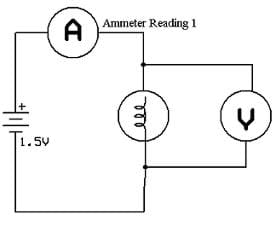Figure 1
2. Next, connect two 1.5 volt batteries in series to the light bulb (see Figure 2). Again, measure the voltage and current, and remember to record your data in the table. Notice the difference in intensity of the light.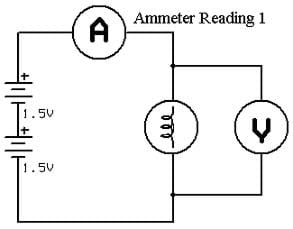Figure 2
3. Connect three 1.5 volt batteries in series (see Figure 3). Once again, measure the voltage and current, and record your data.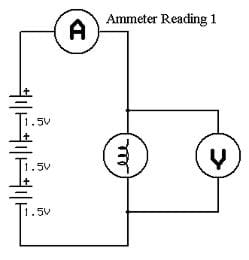Figure 3
4. Now, connect two 1.5 volt batteries in parallel to the bulb (see Figure 4) and measure the voltage and current (again record the data in your table). Is there an increase or decrease in the light's intensity?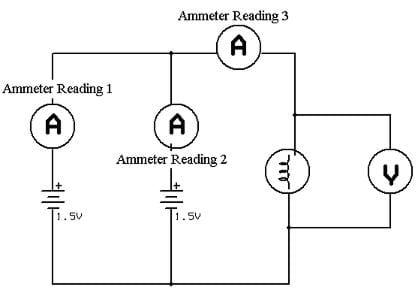Figure 4
5. Connect three 1.5 volt batteries in parallel (see Figure 5). Again, measure and record the voltage and current.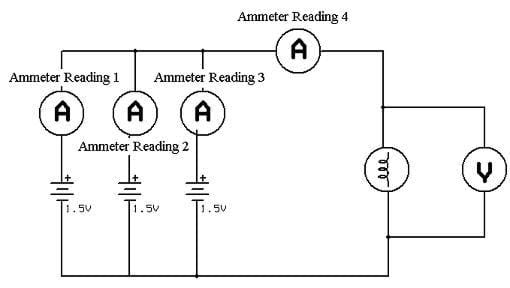Figure 5
6. Comment on the effect of the number of batteries and their arrangement in the circuit on the power produced, and thus on the intensity of the light.

### Vocabulary/Definitions

alternating current: Current that reverses direction at a regular rate.

ammeter: A device that measures current flowing through the circuit.

current: The flow of electrons. Current is read by opening the circuit and connecting the meter in series.

direct current: An electric current that flows in only one direction. The positive and negative terminals of a battery are always, respectively, positive and negative. The current always flows in the same direction between those two terminals.

light Intensity: The amount of light given off by a source such as a light bulb.

load: A device that consumes energy or power.

multimeter: A device that measures the current, voltage and resistance.

parallel circuit: A circuit that has two or more branches for separate currents from one voltage source.

potential: Electrical pressure, also called voltage.

power: The rate at which energy is delivered to something. (quantity / time): Measured in Watts.

resistance: The opposition of a body or substance to current passing through it, resulting in a change of electrical energy into heat, light, or another form of energy. Resistance is measured in Ohms. The resistance of a device is always the same (constant).

series: Circuit that only has one path for the electrons to flow.

voltage: The force that moves electrons. Voltage is read using the meter connected in parallel.

voltmeter: A device that measures the force with which electrons are flowing.

watt: The power expended when one ampere of direct current flows through a resistance of 1 Ohm.

### Assessment

Data Sheet & Questions: After students collect data on their data sheets, assign the questions as homework or a quiz/test. Review their answers to gauge their depth of comprehension.

### Investigating Questions

• How is the brightness of the light bulb affected by the number of batteries connected in series? Explain.
• How is the brightness of the light bulb affected by the number of batteries connected in parallel? Explain.
• How is the current affected by the number of batteries connected in series? Explain.
• How is the current affected by the number of batteries connected in parallel? Explain.
• What are the advantages of connecting the batteries in parallel?
• What are the advantages of connecting the batteries in series?
• How might batteries be connected in a circuit to take advantage of both series and parallel characteristics?

### Safety Issues

• Warn students that light bulbs get hot.
• Use the alligator clips and multimeters with care.

### Troubleshooting Tips

Take multimeter measurements quickly to avoid damage.

### Activity Extensions

Conduct a demonstration that shows students just how much time it takes to use up the "juice" in a battery, and if it is better to use batteries in series or parallel. See the Ohm's Law 2 activity. This may be started before students begin to to work on the Ohm's Law 1 activity.

### Subscribe

Get the inside scoop on all things TeachEngineering such as new site features, curriculum updates, video releases, and more by signing up for our newsletter!
PS: We do not share personal information or emails with anyone.

### More Curriculum Like This

Upper Elementary LessonCircuits: One Path for Electricity

Students learn that charge movement through a circuit depends on the resistance and arrangement of the circuit components. In one associated hands-on activity, students build and investigate the characteristics of series circuits. In another activity, students design and build flashlights.

High School ActivityIntroduction to Circuits and Ohm's Law

Students explore the basics of DC circuits, analyzing the light from light bulbs when connected in series and parallel circuits. Students measure and see the effect of power dissipation from the light bulbs.

High School LessonCircuits

Students are introduced to several key concepts of electronic circuits. They learn about some of the physics behind circuits, the key components in a circuit and their pervasiveness in our homes and everyday lives.

Upper Elementary LessonElectrons on the Move

Students learn about current electricity and necessary conditions for the existence of an electric current. Students construct a simple electric circuit and a galvanic cell to help them understand voltage, current and resistance.